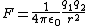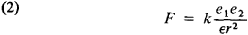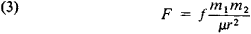Coulomb's law

Also found in: Dictionary, Thesaurus, Medical, Wikipedia.

Coulomb's law

(ko͞o`lŏmz), in physics, law stating that the electrostatic force between two charged bodies is proportional to the product of the amount of charge on the bodies divided by the square of the distance between them. If the bodies are oppositely charged, one positive and one negative, they are attracted toward one another; if the bodies are similarly charged, both positive or both negative, the force between them is repulsive (see chargecharge,
property of matter that gives rise to all electrical phenomena (see electricity). The basic unit of charge, usually denoted by e, is that on the proton or the electron; that on the proton is designated as positive (+e
). Coulomb's law applies exactly only when the charged bodies are much smaller than the distance separating them and therefore can be treated approximately as point charges. When combined with principles of quantum physics, Coulomb's law helps describe the forces that bind electrons to an atomic nucleus, that bind atoms together into molecules, and that hold together solids and liquids. The law was deduced in 1785 by C. A. de CoulombCoulomb, Charles Augustin de
, 1736–1806, French physicist. In 1789 he retired from his posts as military engineer and as superintendent of waters and fountains and devoted himself to continuing his scientific research.
from experimental measures of the forces between charged bodies; the experiments were made using his torsion balance.

Coulomb's law

For electrostatics, Coulomb's law states that the direct force F of point charge q1 on point charge q2, when the charges are separated by a distance r, is given by F = k0q1q2/r2, where k0 is a constant of proportionality whose value depends on the units used for measuring F, q, and r. It is the basic quantitative law of electrostatics. In the rationalized meter-kilogram-second (mks) system of units, k0 = 1/(4 πε0), where ε0 is called the permittivity of empty space and has the value 8.85 × 10-12 farad/m. Thus, Coulomb's law in the rationalized mks system is as in the equation below,where q1 and q2 are expressed in coulombs, r is expressed in meters, and F is given in newtons. See Electrical units and standards

The direction of F is along the line of centers of the point charges q1 and q2, and is one of attraction if the charges are opposite in sign and one of repulsion if the charges have the same sign. For a statement of Coulomb's law as applied to point magnet poles.

Experiments have shown that the exponent of r in the equation is very accurately the number 2. Lord Rutherford's experiments, in which he scattered alpha particles by atomic nuclei, showed that the equation is valid for charged particles of nuclear dimensions down to separations of about 10-12 cm. Nuclear experiments have shown that the forces between charged particles do not obey the equation for separations smaller than this. See Electrostatics

McGraw-Hill Concise Encyclopedia of Physics. © 2002 by The McGraw-Hill Companies, Inc.
The following article is from The Great Soviet Encyclopedia (1979). It might be outdated or ideologically biased.

Coulomb's Law

one of the fundamental laws of electrostatics; determines the force of interaction between two point electric charges at rest—that is, between two electrically charged bodies whose dimensions are small compared with the distance between them. It was established experimentally in 1785 by C. Coulomb using the torsion balance, which he invented.

According to Coulomb's law, two point charges interact with each other in a vacuum with a force F whose magnitude is proportional to the product of the charges e1 and e2 and inversely proportional to the square of the distance r between them:Here k is a proportionality factor that depends on the system of units chosen: in the absolute (Gaussian) system of units (the cgs system), k = 1; in the International System of Units (SI), k = l/47T€o, where eo is the electric constant. The force F is directed along a straight line connecting the charges and is attractive for unlike charges (F < 0) and repulsive for like charges (F > 0).

If the interacting charges are situated in a homogeneous di-electric with dielectric constant €, the force of interaction is then reduced by a factor of ∊:Coulomb's law is one of the experimental foundations of classical electrodynamics; its generalization leads, among other things, to Gauss' theorem.

The law that defines the force of interaction between two magnetic poles is also called Coulomb's law:Here f is a proportionality factor (in the general case it does not coincide with k in the absolute system of units f = 1), m1 and m2 are magnetic charges, and JLI is the magnetic permeability of the medium that surrounds the interacting poles. In a vacuum F = fm1m2/r2.

REFERENCE

Tamm, I. E. Osnovy teorii elektrichestva, 7th ed. Moscow, 1957. Chapter
1, sec. 21; ch. 2, sec. 23.

G. V. VOSKRESENSKII

Coulomb's law

[′kü′lämz ‚lȯ]
(electricity)
The law that the attraction or repulsion between two electric charges acts along the line between them, is proportional to the product of their magnitudes, and is inversely proportional to the square of the distance between them. Also known as law of electrostatic attraction.
McGraw-Hill Dictionary of Scientific & Technical Terms, 6E, Copyright © 2003 by The McGraw-Hill Companies, Inc.
References in periodicals archive ?
Coulomb's Law; the electron, magnetism, magnetic and electric fields
According to Coulomb's law, the shear slip associated with fluid injection is caused by the decrease of normal stress on fracture surface due to an increase of porepressure during fluid injection.
According to Coulomb's law of friction, the relationship among shear stress, [tau], normal stress, [[sigma].sub.N], the coefficient of friction, [f.sub.S], and pore-pressure, [P.sub.P], within a fracture surfaces at the moment of shear slip (critical condition) due to an increase in pore-pressure are described as follow,
The friction coefficients [Mu] = 0.02 (Coulomb's law) and [Mathematical Expression Omitted] (Tresca's law) are low but similar to those deduced by Makinouchi (22) from quasi-static experiments.
According to this simple proposal, bodies obey the law of gravity and Coulomb's law, cp, but cetera just aren't paria with respect to the former law when the bodies in question have significant charge; and other things are not equal with respect to the latter law when the quanta in question are affected by nearby dense masses.
The ideal gas law would have to be understood in terms of what would happen if molecules took up no space and failed to be subsumed by Coulomb's law. Or consider a particularly striking example from astrophysics: Dicke, Peebles, et al.
The force [[??].sub.QQ] represents Coulomb's law for the electromagnetic interaction between two electric charges.
Newton's law of gravity and Coulomb's law of electric force are classically unified into a single expression of the interaction between the complex energies of two electrically charged particles.
It is well known that mass and electric charge are two fundamental properties (inertia and electricity) of matter, which directly determine the gravitational and electromagnetic interactions via Newton's law of gravity  and Coulomb's law of electric force .

Site: Follow: Share:
Open / Close